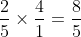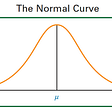# Types of Probability Distributions

A probability distribution is a statistical function that describes all the possible values and likelihoods that a random variable can take within a given range. This range will be bounded between the minimum and maximum possible values, but precisely where the possible value is likely to be plotted on the probability distribution depends on a…

--

--

--

## More from Jagandeep Singh

Love podcasts or audiobooks? Learn on the go with our new app.

## Division with fractions why multiply with reciprocal## Cracking the Medium Code — Day 3## Grover’s Algorithm: Quantum Algorithms Untangled## What does “randomness” truly mean?## The Assumptions in Linear Regression## Normal Distribution: Most Widely Used Distribution## Are A and B Independent?